﻿ Digital Circuits and Systems - Circuits i Sistemes Digitals (CSD) - EETAC - UPCBachelor's Degree in Telecommunications Systems and in Network Engineering## Circuits based on logic gates

1. Analysis specifications

Deduce the truth table of circuit in Fig. 1 using the four methods stated in lecture .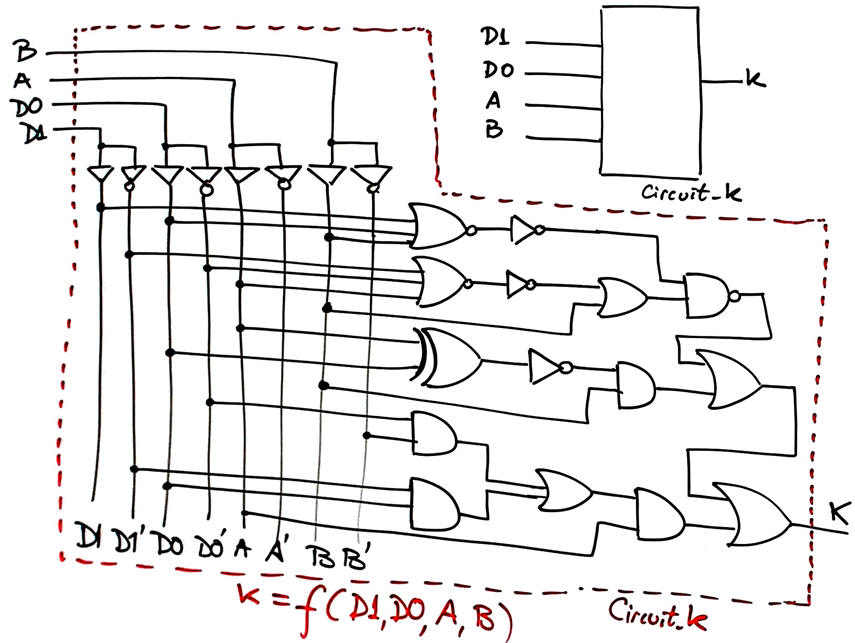Fig. 1. Example Circuit_K to analyse.

2. Planning how to analyse the circuit

• Method I: Proteus

• Method II: WolframAlpha

• Method III: pen & paper and Boolean algebra.

• Method IV: VHDL tools for synthesis and simulationFig. 2. Analysis concept map.

Place the projects in different folders and name them accordingly. Normally we use our C: hard disk drive, for instance:

C:\CSD\P1\Circuit_K\Proteus\Circuit_K.pdsprj (the circuit captured in Proteus to  be simulated)

C:\CSD\P1\Circuit_K\Wolfram\Circuit_K.txt  (logic equations compatible with WolframAlpha engine)

C:\CSD\P1\Circuit_K\Algebra\Circuit_K.jpg, circuit_K.pdf, etc. (pictures, scanned sheets of paper, ...)

C:\CSD\P1\Circuit_K\VHDL\Circuit_K.vhd, Circuit_K_tb.vhd  (VHDL files for running the EDA tools)

Furthermore, something very important: do not start a project or a simulation from scratch but copying and adapting a similar exercise or file from this web. For instance Circuit_W in lecture or from the highlighted project Circuit_C. Our web contains many examples and exercises that can be used as templates to copy and adapt.

3. Analysisi development

Developing means doing it, executing a given plan to achieve a solution.

Analysis:   The way to proceed and the necessary CAD/EDA tools will depend on the path you follow. For example:

Method I.- Circuit simulator: Draw/capture  the circuit schematic in Proteus and run a simulation.

Try all the possible input combinations to complete the truth table. Use, copy and adapt files from . This is an example circuit captured: Circuit_K.pdsprj.Fig. 3. This is the solution of the truth table for a given input stimulus vector. You can repeat 2^4 = 16 times to measure and an write down the complete truth table.

Method II.- Numerical engine WolframAlpha.

Deducing the circuit's equation analysing the circuit.Fig. 4. Circuit and equation.Write the circuit equation in a text file step by step, for instance, Circuit_K equation text. Copy and paste it in WolframAlpha and run the engine to obtain the circuit's truth table. pay attention to the variable order before annotating minterns or maxterms.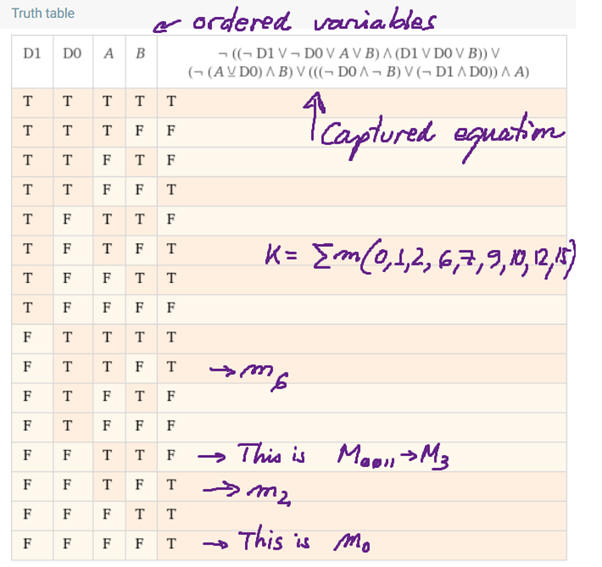Fig. 5. WolframAlpha results from the circuit's equation in Fig. 4.

Method III.- Analytical method using Boole's algebra.

When solving a circuit in paper, this is the starting point: draw Circuit_K from Fig. 1 and analyse it to deduce its equivalent equation as it was done in Fig. 4. Proceed using Boolean algebra following the plan until you obtain a simplified expression like SoP or PoS.

Discuss results with your group mates, and continue adding missing variables until your products are transformed into minterms, or your sums into maxterms.

There are some notes and on the analysis of Circuit_K using method III.

Method IV.- VHDL project

Write a VHDL file like this Circuit_K.vhd consisting of entity and architecture based on the circuit's algebraic equation shown in Fig. 4.

Start a VHDL synthesis project using an EDA tool for a given target chip, such MAX II EPM2210F324C3 CPLD from Intel.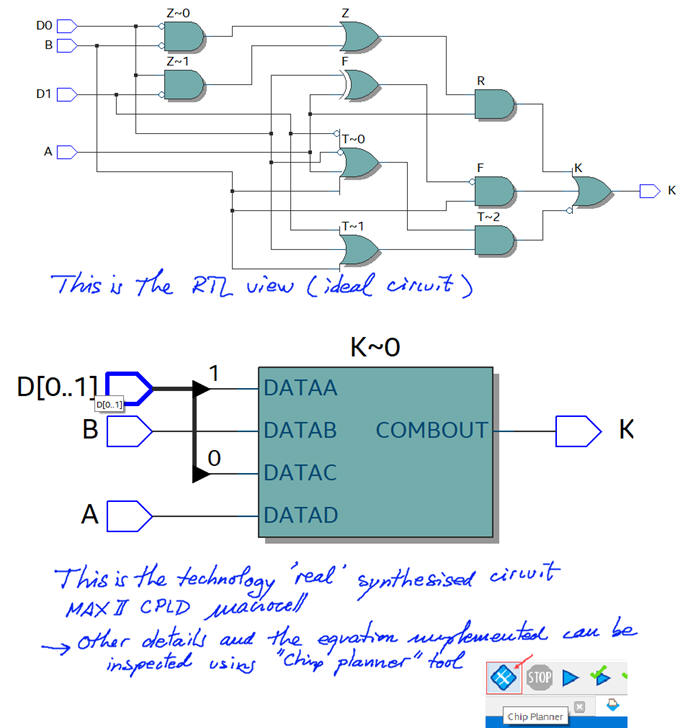Fig. 6. RTL and technology results

Once examined the RTL and technology views, continue starting a VHDL simulation project using a testbench to obtain the circuit's truth table. This is an example testbench Circuit_K_tb.vhd file written translating all truth table combinations into the skeleton generated from Quartus Prime.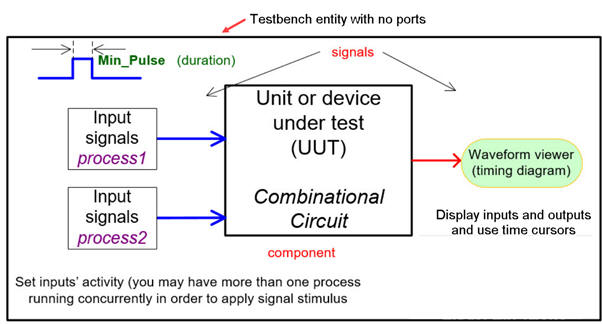Fig. 7. VHDL testbench fixture for measuring how the circuit works using a timing diagram when applying several input stimulus.

In this analysis project, simulation all truth table combinations will allow us complete the circuit's truth table.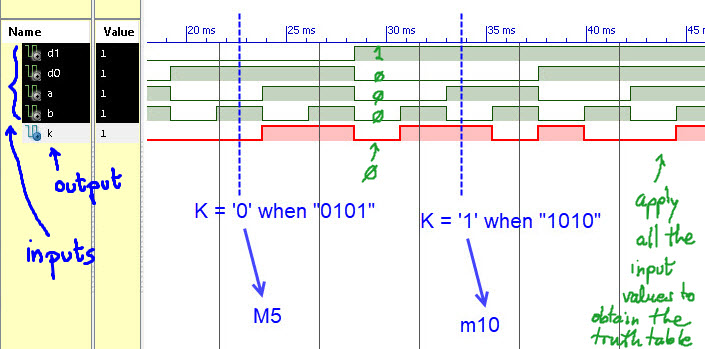Fig. 8. Example of a simulation using VHDL tools of the Circuit_K.  Testing all the combinations means obtaining the truth table of the circuit as it was done using the method I in  Proteus.

4. Testing results

In this analysis problem, the way to check the truth table deduced from one method is by comparison with the truth table obtained by other methods. You can compare solutions with your team mates.

5. Analysisi reports

This pdf report shows Circuit_K solved using Method IV and verification using Method II.